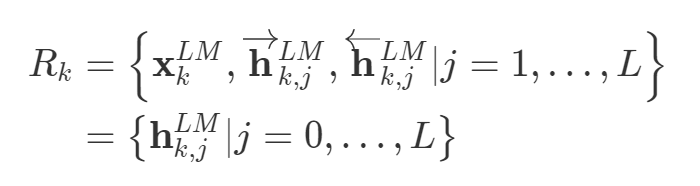# 词向量-ELMo介绍

Deep contextualized word representations获得了NAACL 2018的outstanding paper award，其方法有很大的启发意义，本文则是对其做了一个简要梳理。

## Motivation

• 预训练的word representation在NLP任务中表现出了很好的性能，已经是很多NLP任务不可或缺的一部分，论文作者认为一个好的word representation需要能建模以下两部分信息：
• 单词的特征，如语义，语法
• 单词在不同语境下的变化，即一词多义
• 之前很多工作的word representation是固定的，如word2vec/glove等word representation对于一个词，其向量是固定的，无法很好的处理一词多义的情况

## Introduction

• 在大规模语料中利用bidirectional LSTM模型训练语言模型（language model），ELMo（Embedding from language model）也由此得名
• 为了应用到下游任务，利用下游任务的语料库（无需label）对预训练的语言模型进行微调，然后再利用下游任务有标注的数据进行supervised learning

• Embedding是“deep”的，这体现在ELMo是biLM（bi-Language model）内部所有层的综合，这样可以有充分的信息来建模word representation：
• Higher-level LSTM states可以捕获上下文相关的语义信息，不用修改就可以用来做词义消歧
• Lower-level LSTMstates 可以捕获语法相关的信息，可以用作词性标注任务
ELMo综合了上述信息，而之前的工作只利用了top LSTM layer的信息
• 其词向量是动态的，即根据当前上下文环境来产生当前词向量，而不是一个固定的外部词向量

## Bidirectional language model

• 前向语言模型：从历史信息预测下一个词，即从给定的历史信息\left(t_{1}, \ldots, t_{k-1}\right)建模下一个token t_k的概率
p\left(t_{1}, t_{2}, \ldots, t_{N}\right)=\prod_{k=1}^{N} p\left(t_{k} | t_{1}, t_{2}, \ldots, t_{k-1}\right)
对于一个L层的LSTM，设其输入是x_k^{LM} (token embedding)，每一层都会输出一个context-dependent representation \overrightarrow \mathbf{h}_{k, j}^{L M}，LSTM的最后一层输出为\overrightarrow \mathbf{h}_{k, L}^{L M}，该输出会在Softmax layer被用来预测下一个token t_{k+1}
• 后向语言模型：从未来信息预测上一个词，即从给定的未来\left(t_{k+1}, \ldots, t_{N}\right)建模上一个token t_k的概率
p\left(t_{1}, t_{2}, \ldots, t_{N}\right)=\prod_{k=1}^{N} p\left(t_{k} | t_{k+1}, t_{k+2}, \ldots, t_{N}\right)
同样地，对于一个L层的LSTM，设其输入是x_k^{LM} (token embedding)，每一层都会输出一个context-dependent representation \overleftarrow \mathbf{h}_{k, j}^{L M}，LSTM的最后一层输出为\overleftarrow \mathbf{h}_{k, L}^{L M}，该输出会在Softmax layer被用来预测上一个token t_{k-1}

\begin{array}{l}{\sum_{k=1}^{N}\left(\log p\left(t_{k} | t_{1}, \ldots, t_{k-1} ; \Theta_{x}, \vec{\Theta}_{L S T M}, \Theta_{s}\right)\right.} \\ {\left.\quad+\log p\left(t_{k} | t_{k+1}, \ldots, t_{N} ; \Theta_{x}, \overleftarrow \Theta_{L S T M}, \Theta_{s}\right)\right)}\end{array}

• \Theta_{x}是token embedding
• \Theta_{s}代表softmax layer的参数

biLM利用了biLSTM，biLSTM在前向和后向两个方向上共享了部分权重

## ELMo• \mathbf{h}_{k, 0}^{L M}是token layer
• \mathbf{h}_{k, j}^{L M}=\left[\overrightarrow{\mathbf{h}}_{k, j}^{L M} ; \overleftarrow{\mathbf{h}}_{k, j}^{L M}\right], 代表biLSTM layer

\mathbf{E L M o}_{k}^{t a s k}=E\left(R_{k} ; \Theta^{t a s k}\right)=\gamma^{t a s k} \sum_{j=0}^{L} s_{j}^{t a s k} \mathbf{h}_{k, j}^{L M}

1. 直接将ELMo词向量ELMo_k和普通词向量x_k拼接得到\left[\mathbf{x}_{k} ; \mathbf{E L M o}_{k}^{t a s k}\right]作为model输入
2. 将ELMo词向量ELMo_k和隐藏层输出h_k进行拼接得到\left[\mathbf{h}_{k} ; \mathbf{E} \mathbf{L} \mathbf{M} \mathbf{o}_{k}^{t a s k}\right]

## Evaluation

• Textual entailment:
stanford natural language inference (SNLI)数据集上提升了1.4%。
• Semantic role labeling:
比之前的state-of-the-art模型提高了3.2%，将ELMo加入到之前的state-of-the-art的单模型中，提升了1.2%。
• Coreference resolution:
比之前的state-of-the-art模型提高了3.2%，将ELMo加入到之前的state-of-the-art的ensemble模型中，提升了1.6%。
• Named entity extraction:
• Sentiment analysis:
比之前的state-of-the-art模型提高了3.3%，将ELMo加入到之前的state-of-the-art模型中，提升了1%。

## 如何在代码中使用ELMo

### 使用ELMo预训练模型

pip install allennlp

TensorFlow：

Pytorch: